# Running Mathematica within a batch environment

Mathematica can easily used within a batch environment, this is without user interaction. This is desirable e.g. if Mathematica is used for the the estimation of market parameters every few hours.

Information on how to do this is scattered over "The Mathematica book" and we were asked several times about this feature. The following example will demonstrate the use of Mathematica in a batch environment under Microsoft windows. The same should also be available with other operating systems.

To simplify the typing and to allow Mathematica to find the necessary files make sure that the currend directory is the root directory for Mathematica. On most installations using the command Directory  right after the start of the Mathematica front end should return the root directory.

````
``

Now we simply create a simple text file which will hold the Mathematica commands we want to have evaluated

``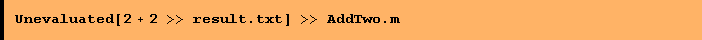``

Unevaluated avoids the immidiate evaluation of this expressions. The newly created file now looks like the following.

``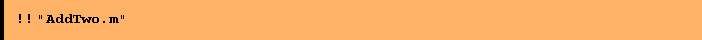``
`2 + 2 >> "result.txt"`

All text editors can be used to create such a file. There is no need to use Mathematica for creating the text file. To let the Mathematica Kernel evaluate the commands in the input file just use a DOS shell, move to the root directory and type in

as shown below: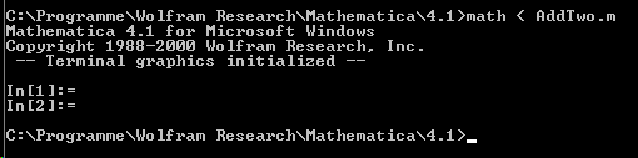In an batch environment the last line would be part of an *.bat or other scripting file.
The same can be achieved from within Mathematica using the Run command.

``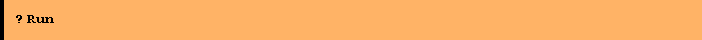``
``
````
`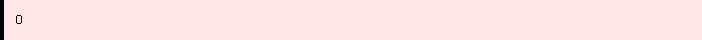`

The result of this can be found in the file result.txt.

``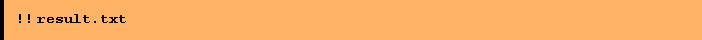``
`4`

The same approach works with Mathematica notebooks. They are also text based and readily interpretable for the Mathematica kernel. To demonstrate this evaluate the following Mathematica lines. First create a new notebook, either by using the front end menu File|New typing as an example

4+4>> result.txt

into the newly created notebook and save it as "AddTwo.nb" in the root directory. Alternativly one can evaluate the following lines, which let the Kernel perform the described steps

``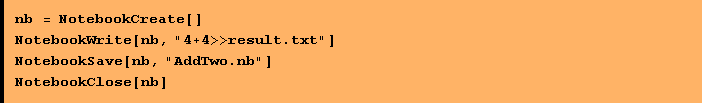``
`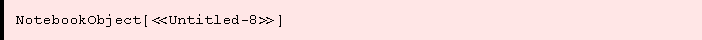`

To see how the Mathematica Kernel works on this notebook use the DOS shell again, move to the Mathematica root directory and type this time.

This will write the output file with the following content.

````
`8`

For logging purposes it might be usefull to catch error or other messages generated from Mathematica. This can be achieved through providing another parameter on the command line.